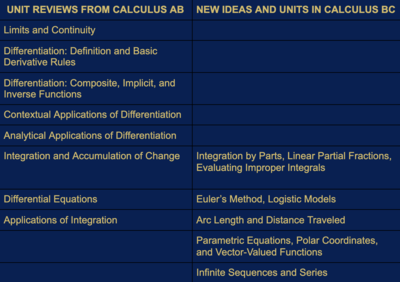# US Math

•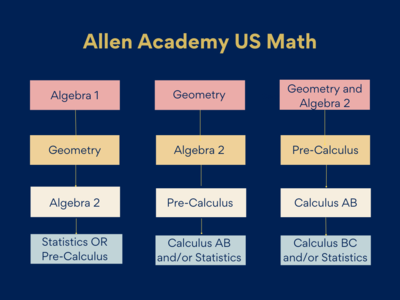Algebra 1

In Algebra I the fundamental purpose of this course is to formalize and extend the mathematics that students learned in the middle grades. Instruction will focus on five critical areas: (1) analyze and explain the process of solving equations and inequalities: (2) learn function notation and develop the concepts of domain and range; (3) use regression techniques; (4) create quadratic and exponential expressions; and (5) select from among these functions to model phenomena. Each critical area is described below.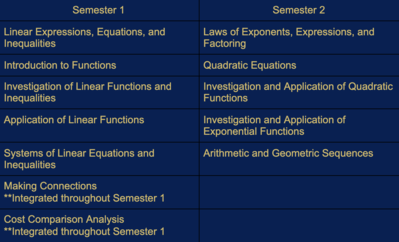Geometry

A comprehensive look at the study of geometric concepts including the basic elements of geometry, proofs, parallel and perpendicular lines, the coordinate plane, triangles, quadrilaterals, polygons, circles, trigonometry, congruence and similarity, surface area, volume and transformations.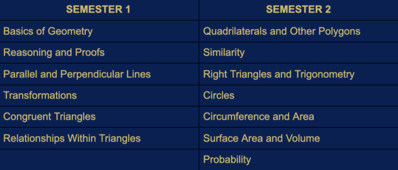Algebra 2

Algebra 2 advances the students’ understanding of functions, starting with linear functions and progressing onward to quadratics, rational functions, polynomials, exponential and logarithmic functions. Matrices, exponents, factoring, and algebraic fractions are also explored in detail. Graphing and graph transformations are a fundamental part of the course. Real-world modeling with data enhances their appreciation of the types of functions studied. Students are expected to make connections between algebraic and graphical approaches to solving problems. Students will learn technology that complements their conceptual understanding, such performing linear regressions or finding intersections of graphs.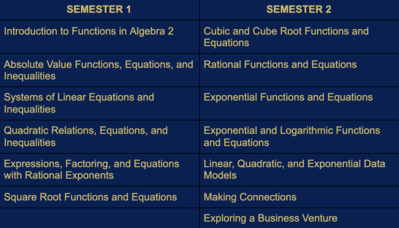Statistics

The statistics course introduces students to the major concepts and tools for collecting, analyzing and drawing conclusions from data. Students are exposed to four broad conceptual themes: 1. Exploring Data: Describing patterns and departures from patterns 2. Sampling and Experimentation: Planning and conducting a study 3. Anticipating Patterns: Exploring random phenomena using probability and simulation 4. Statistical Inference: Estimating population parameters and testing hypotheses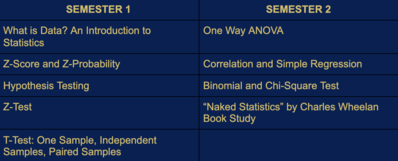PreCalculus

Precalculus is the preparation for calculus. The course approaches topics from a function point of view, where appropriate, and is designed to strengthen and enhance conceptual understanding and mathematical reasoning used when modeling and solving mathematical and real-world problems. Students systematically work with functions and their multiple representations. The study of Precalculus deepens students' mathematical understanding and fluency with algebra and trigonometry and extends their ability to make connections and apply concepts and procedures at higher levels. Students investigate and explore mathematical ideas, develop multiple strategies for analyzing complex situations, and use technology to build understanding, make connections between representations, and provide support in solving problems.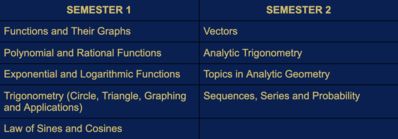AP Calculus AB

AP Calculus AB is the study of limits, derivatives, definite and indefinite integrals, and the Fundamental Theorem of Calculus. Consistent with AP philosophy, concepts will be expressed and analyzed geometrically, numerically, analytically, and verbally.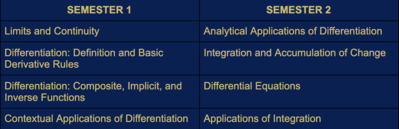AP Calculus BC

This is a full-year course in the calculus of functions of a single variable. It includes all topics covered in Calculus AB plus additional topics. AP Calculus BC is the study of limits, derivatives, definite and indefinite integrals, polynomial approximations and (infinite) series. Though this is considered a study of single-variable calculus, parametric, polar, and vector functions will be studied. Consistent with AP philosophy, concepts will be expressed and analyzed geometrically, numerically, analytically, and verbally. Calculus BC covers topics that are usually included in the first 3 semesters of college calculus.